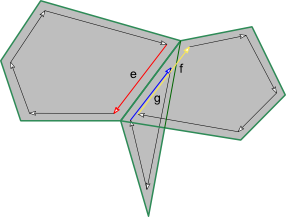# Half-edges

VEX has functions that let you treat edges as unshared per-face “half-edges”.

## Overview ¶In Houdini, edges are normally treated as undirected and shared between faces. However, for some tasks (such as simplifying or cleaning geometry) it is useful to view faces as each having their own edges.You can think of this as splitting each shared undirected edge along its length into two half edges. (Boundary edges of course will only have one “half-edge”.)Each half-edge is directed (it has a start vertex and an end vertex).The half-edge functions let you quickly find a half-edge’s source and destination vertex, the next half-edge, get the other half-edges from the same edge, find all half-edges sharing a given point, and other manipulations.

## Equivalence ¶

Two half-edges are equivalent if they are “split” from the same shared edge. In the following figure, three primitives share an edge. The half-edges e, f, and g are equivalent because they are from the same edge. Directionality does not matter – e is considered equivalent to f and g even though they have opposite directions.The source (and destination) vertex of a half-edge uniquely identify it, meaning that there can be at most one half-edge with a given vertex as the source. This is because a vertex can belong to only one primitive. However, because several vertices can be wired to the same point, half-edges can have shared source and destination points. Another way of thinking about equivalence is that two half-edges are equivalent if their vertices are shared by the same two points.

One of a set of equivalent half-edges is considered the primary half-edge in that class. The VEX functions let you check if a given half-edge is primary, or given a non-primary half-edge, find the equivalent primary half-edge. In the case of an unshared edge, it only has a single primary half-edge.

## Functions ¶

 Check whether a half-edge is valid Check whether a half-edge is primary Find the primary equivalent half-edge. If it belongs to an unshared edge, then the same half-edge is returned. Test whether two half-edges are equivalent Iterate over the half-edges in an equivalence class Get the number of half-edges in an equivalence class Get the source vertex of a half-edge Get the destination vertex of a half-edge Get the vertex preceding the source vertex in half-edge primitive Get the vertex following the destination vertex in half-edge primitive Get the primitive of a half-edge Get the source point of a half-edge Get the destination point of a half-edge Get the pre-source point of a half-edge Get the post-destination point of a half-edge Get the next half-edge in primitive Get the previous half-edge in primitive Find half-edge between two points Find half-edge with given source and destination Find a half-edge with a given source point Iterate over half-edges with a given source point Get the half-edge with a source vertex Get a half-edge on a given primitive

# VEX

## Language ¶

• Details of VEX syntax, data types, and so on.

• Overview of how to use the VEX language compiler vcc and its pre-processor and pragma statements.

## Next steps ¶

• You can read the contents of primitive/point/vertex groups in VEX as if they were attributes.

• Examples and suggestions for programming in VEX.

• VEX has functions that let you treat edges as unshared per-face half-edges.

• You can use the assert() macro to print information while you are debugging VEX code.

## Reference ¶

• Guide to the different contexts in which you can write VEX programs.